Browse by Topic
Related Topics

# How to Win at Coin Flipping

Let’s flip a coin, over and over. Beforehand, the players each pick a sequence of flips. The sequence that occurs first wins. With HH vs. TH, HH will win if the first two flips are HH and will lose if any of those flips are tails. HH vs. TH has a 1/4 vs. 3/4 possibility of winning.

Phrased a different way: Suppose I offer a bet on a series of coin flips. One of these bets would be bad for you. Which one? The odds of the event occurring are given at the end. (The second bet is the bad bet to take.)

HTT appears before TTT. If it does, I give you \$1. If not, you give me \$4. (7/8)
HHT appears before TTT. If it does, I give you \$1. If not, you give me \$3. (7/10)
THH appears before HHT. If it does, I give you \$1. If not, you give me \$2. (3/4)
HTH appears before THH. If it does, I give you \$1. If not, you give me \$1. (1/2)

This is the strange world of Penney’s game. Here is a table of odds and facts for various 3-flip games. Calculating these odds can be both tedious and mathematically demanding—a natural job for Mathematica or Mathematica Home Edition.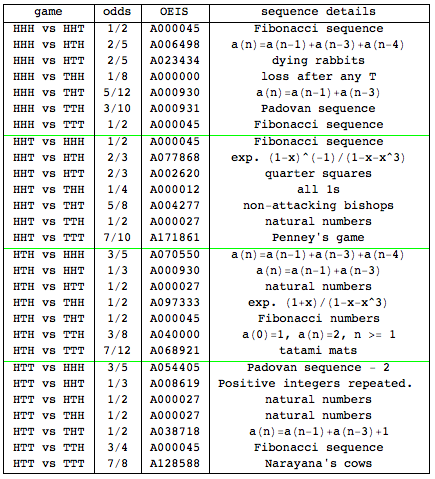As a picture, here are the 6 games that are not obvious losers. For any game your opponent picks, you can pick a game that beats it, making this a nontransitive game.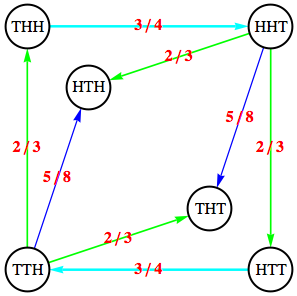The first game is HHH vs. HHT. Here are the ways HHH can win after 3, 4, 5, and 6 flips. Notice that each win ends HHH. The corresponding number of wins is the beginning of the Fibonacci sequence.

 HHH 1 THHH 1 HTHHH TTHHH 2 HTTHHH THTHHH TTTHHH 3

The sequences of a game can be calculated with the piece of code in the downloadable Computable Document Format (CDF) file. The set of flips that could have a winner is calculated, and then all the winners are removed. A head and a tail are added to all games without a winner, and then the process is iterated.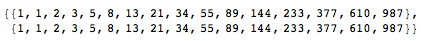With the sequence, FindSequenceFunction or FindGeneratingFunction can be used to get the underlying function. The previous example might be too easy.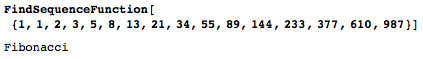Here’s a harder sequence. The sequence from the brute-force code leads to a generating function, which can be used to find many more terms in the sequence.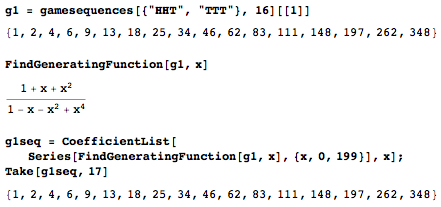Again, the start of a sequence was found by brute-force methods, and that sequence led to an elegant function. From that, many more terms were calculated. With results from games of 200 flips or less, the odds can be determined very precisely. The odds of HHT beating TTT are 7/10.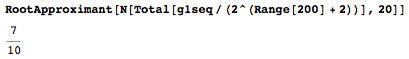Repeating all of this for the 4-flip games, here are the 14 games with non-obvious strategies. HHHH and TTTT are losers, from an odds standpoint. Notice the clockwise ring of arrows on the outside. Every game tends to beat the game in front of it.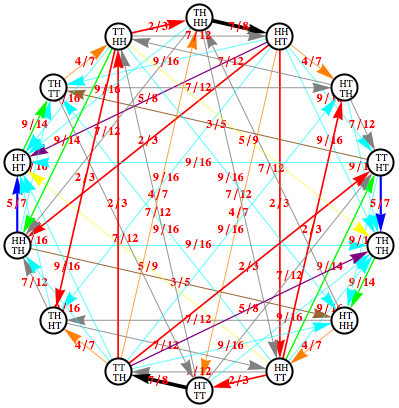One 4-flip game with strange odds is HHHH vs. TTTH. The fraction 22/7 is a fairly good approximation for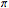, so the odds of a win are about 1/.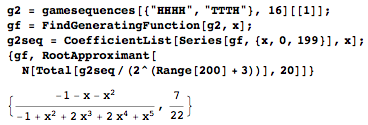A few years ago, I tried to present this problem quickly, and ended up getting the math wrong. I only looked at games up to a certain short length, and then approximated the odds from there. But that was the wrong way to do it, and the odds were all off. Fortunately, someone pointed out my mistake, and this world of strange games opened up for me as I harnessed the full power of Mathematica.

If just the odds are wanted instead of the sequences, there is an easier way, developed by John Conway.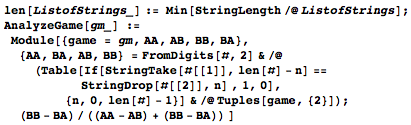The algorithm quickly calculates a table of values.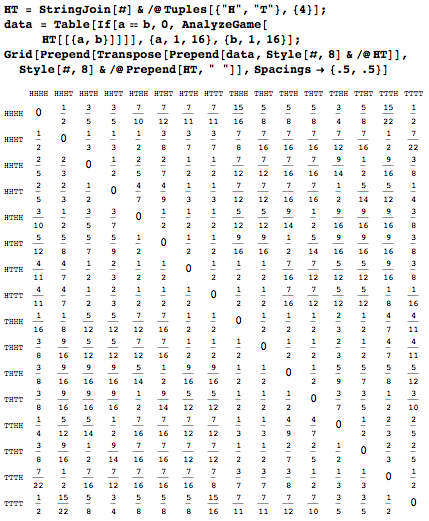Even a grid of all the 8-flip games can be easily calculated.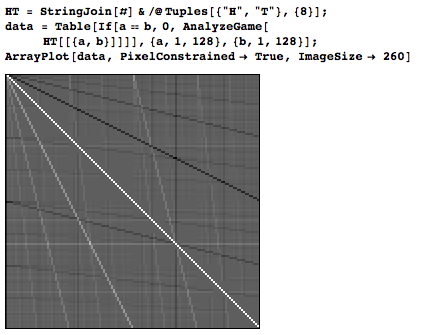So, which do you prefer out of HHHTT and HTTHH? One of them wins 7/13 of the time, and the other 6/13. Figuring out which is which requires some powerful tools.